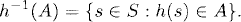# MEASURABLE FUNCTIONS

In measure theory, measurable functions are defined by the property of their pre-images. The definition of measurable functions is similar to the definition of continuous functions in topology. In this chapter, we introduce measurable functions and relevant theorems.

# 5.1 Basic Concepts and Facts

Definition 5.1 (∑-Measurable Function). Let (S, ∑) be a measurable space. A function h : SR is called ∑-measurable, or measurable relative to the σ-algebra ∑, if and only ifwhereis the Borel σ-algebra on R (see Definition 2.6) and h−1(A) is defined asThe set of all ∑-measurable functions is denoted by m∑.

Definition 5.2 (Borel Function). Let S be a topological space (see Definition 2.5). A function h : SR is called a Borel function if h is B(S)-measurable or Borel measurable, where(S) is the σ-algebra generated by the collection of all open sets in S.

Definition 5.3 (∑1/∑2-measurable Function). Let (S1, ∑1) and (S2, ∑2) be two measurable spaces and h : S1S2. h is considered ∑1/∑2-measurable if and only if

where h−1(A) = {s S1 : h(s) A}.

Definition ...

Get Measure, Probability, and Mathematical Finance: A Problem-Oriented Approach now with the O’Reilly learning platform.

O’Reilly members experience live online training, plus books, videos, and digital content from nearly 200 publishers.